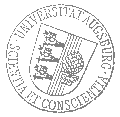# Universität AugsburgInstitut für MathematikOberseminar zu Differentialgleichungen

Dr. Jonas Tölle
Universität Helsiniki

spricht am

Donnerstag, 23. Januar 2020

um

15:45 Uhr

im

Raum 2004 (L1)

über das Thema:

## »The stochastic Klausmeier system and a stochastic Schauder-Tychonoff type theorem«

 Abstract: Pattern formation at the ecosystem level is a rapidly growing area of spatial ecology. Theoretical models are a widely used tool for studying e.g. banded vegetation patterns. One important model is the system of advection-diffusion equations proposed by Klausmeier which is a model for vegetation dynamics in semi-deserted areas. It is a generalization of the so-called Gray-Scott system which already exhibits effects similar to Turing patterns. The underlying mathematics of this model is given by a pair of solutions \$(u,v)\$ to a partial differential equation system coupled by a nonlinearity. The function \$u\$ represents the surface water content and \$v\$ represents the biomass density of the plants. In order to model the spread of water on a terrain without a specific preference for the direction in which the water flows, the original models were extended by replacing the diffusion operator by a nonlinear porous media operator, which represents the situation that the ground is partially filled by interconnected pores conveying fluid under an applied pressure gradient.

 Hierzu ergeht herzliche Einladung. Prof. Dr. Dirk Blömker

[Impressum]      [Datenschutz]      wwwadm@math.uni-augsburg.de,     Fr 6-Dez-2019 10:27:54 MEZ